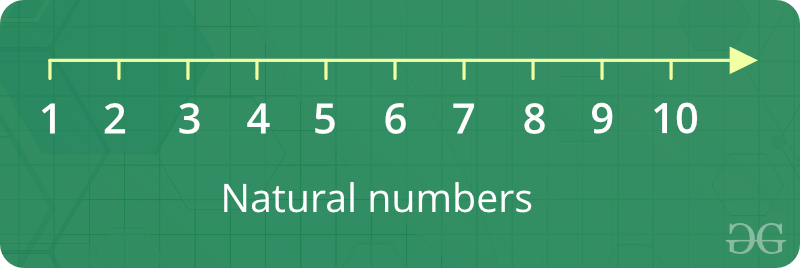# Natural Numbers

Counting numbers like 1, 2, 3, 4, 5, 6 … Basically, all integers greater than 0 are natural numbers.1. They are whole numbers (called integers), and never less than zero (i.e. positive numbers)
2. The next possible natural number can be found by adding 1 to the current natural number
3. The natural numbers are the ordinary numbers, 1, 2, 3, etc., with which we count.
4. The number zero is sometimes considered to be a natural number.Not always because no one counts starting with zero, 0, 1, 2, 3.
5. GCD of all other natural numbers with a prime is always one.
6. The natural numbers can be defined formally by relating them to sets. Then, zero is the number of elements in the empty set; 1 is the number of elements in the set containing one natural number; and so on.

How to print sum of n natural Numbers ?

Using Recursion

Given a number n, find sum of first n natural numbers. To calculate the sum, we will use a recursive function `recur_sum()`.

Examples :

```Input : 3
Output : 6
Explanation : 1 + 2 + 3 = 6

Input : 5
Output : 15
Explanation : 1 + 2 + 3 + 4 + 5 = 15
```

## C++

 `// C++ program to find the  ` `// sum of natural numbers up ` `// to n using recursion ` `#include ` `using` `namespace` `std; ` ` `  `// Returns sum of first  ` `// n natural numbers ` `int` `recurSum(``int` `n) ` `{ ` `    ``if` `(n <= 1) ` `        ``return` `n; ` `    ``return` `n + recurSum(n - 1); ` `} ` ` `  `// Driver code ` `int` `main() ` `{ ` `    ``int` `n = 5; ` `    ``cout << recurSum(n); ` `    ``return` `0; ` `} `

## Java

 `// Java program to find the  ` `// sum of natural numbers up ` `// to n using recursion ` `import` `java.util.*; ` `import` `java.lang.*; ` ` `  `class` `GFG ` `{ ` ` `  `    ``// Returns sum of first  ` `    ``// n natural numbers ` `    ``public` `static` `int` `recurSum(``int` `n) ` `    ``{ ` `        ``if` `(n <= ``1``) ` `            ``return` `n; ` `        ``return` `n + recurSum(n - ``1``); ` `    ``} ` `     `  `    ``// Driver code ` `    ``public` `static` `void` `main(String args[]) ` `    ``{ ` `        ``int` `n = ``5``; ` `        ``System.out.println(recurSum(n)); ` `    ``} ` `} `

## Python

 `# Python code to find sum  ` `# of natural numbers upto ` `# n using recursion ` ` `  `# Returns sum of first ` `# n natural numbers ` `def` `recurSum(n): ` `    ``if` `n <``=` `1``: ` `        ``return` `n ` `    ``return` `n ``+` `recurSum(n ``-` `1``) ` ` `  `# Driver code ` `n ``=` `5` `print``(recurSum(n)) `

## C#

 `// C# program to find the  ` `// sum of natural numbers ` `// up to n using recursion ` `using` `System; ` ` `  `class` `GFG ` `{ ` ` `  `    ``// Returns sum of first  ` `    ``// n natural numbers ` `    ``public` `static` `int` `recurSum(``int` `n) ` `    ``{ ` `        ``if` `(n <= 1) ` `            ``return` `n; ` `        ``return` `n + recurSum(n - 1); ` `    ``} ` `     `  `    ``// Driver code ` `    ``public` `static` `void` `Main() ` `    ``{ ` `        ``int` `n = 5; ` `        ``Console.WriteLine(recurSum(n)); ` `    ``} ` `} `

## PHP

 ` `

Output :

`15 `

Using Loop

A simple solution is to do following.

```1) Initialize : sum = 0
2) Run a loop from x = 1 to n and
do following in loop.
sum = sum + x ```

## C++

 `// CPP program to find sum of first ` `// n natural numbers. ` `#include ` `using` `namespace` `std; ` ` `  `// Returns sum of first n natural ` `// numbers ` `int` `findSum(``int` `n) ` `{ ` `   ``int` `sum = 0; ` `   ``for` `(``int` `x=1; x<=n; x++)  ` `     ``sum = sum + x; ` `   ``return` `sum; ` `} ` ` `  `// Driver code ` `int` `main() ` `{ ` `  ``int` `n = 5; ` `  ``cout << findSum(n); ` `  ``return` `0; ` `}  `

## Java

 `// JAVA program to find sum of first ` `// n natural numbers. ` `import` `java.io.*; ` ` `  `class` `GFG{ ` ` `  `    ``// Returns sum of first n natural ` `    ``// numbers ` `    ``static` `int` `findSum(``int` `n) ` `    ``{ ` `        ``int` `sum = ``0``; ` `        ``for` `(``int` `x = ``1``; x <= n; x++)  ` `            ``sum = sum + x; ` `        ``return` `sum; ` `    ``} ` ` `  `    ``// Driver code ` `    ``public` `static` `void` `main(String args[]) ` `    ``{ ` `        ``int` `n = ``5``; ` `        ``System.out.println(findSum(n)); ` `    ``}  ` `} `

## Python

 `# PYTHON program to find sum of first ` `# n natural numbers. ` ` `  `# Returns sum of first n natural ` `# numbers ` `def` `findSum(n) : ` `    ``sum` `=` `0` `    ``x ``=` `1` `    ``while` `x <``=``n : ` `        ``sum` `=` `sum` `+` `x ` `        ``x ``=` `x ``+` `1` `    ``return` `sum` ` `  ` `  `# Driver code ` ` `  `n ``=` `5` `print` `findSum(n) `

## C#

 `// C# program to find sum of first ` `// n natural numbers. ` `using` `System; ` ` `  `class` `GFG{ ` ` `  `    ``// Returns sum of first n natural ` `    ``// numbers ` `    ``static` `int` `findSum(``int` `n) ` `    ``{ ` `        ``int` `sum = 0; ` `        ``for` `(``int` `x = 1; x <= n; x++)  ` `            ``sum = sum + x; ` `        ``return` `sum; ` `    ``} ` ` `  `    ``// Driver code ` `    ``public` `static` `void` `Main() ` `    ``{ ` `        ``int` `n = 5; ` `        ``Console.Write(findSum(n)); ` `    ``}  ` `} `

## PHP

 ` `

Output :

```15
```

Using Sum of n terms formula

Formula for finding sum of n natural numbers is given by n*(n+1)/2 which implies if the formula is used the program returns output faster than it would take iterating over loop or recurrsion. Time complexity is O(1).